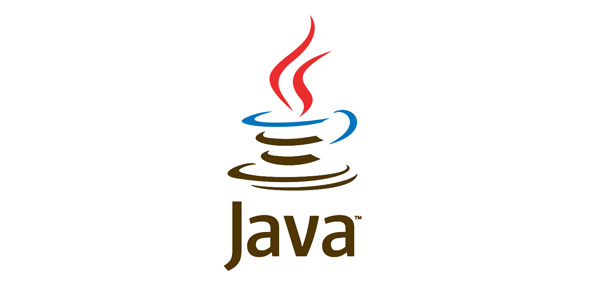# Java Fundamental Mock Exam

20 Questions | Total Attempts: 142SettingsSample Java Quiz

• 1.
All Java source files must end with the extension .class
• A.

True

• B.

False

• 2.
A source file may contain an unlimited number of non-public class definitions.
• A.

True

• B.

False

• 3.
If a source file includes a public class, the class name must match the unextended filename.
• A.

True

• B.

False

• 4.
Each source file that you compile will produce one file with an extension .class
• A.

True

• B.

False

• 5.
At least one of the following three top-level elements must appear in every source file.  If they are included in the source file, they must appear in the following order.A.  package declaration B.  import declarations C.  class definitions
• A.

True

• B.

False

• 6.
The compiler does not require you to use import directives.
• A.

True

• B.

False

• 7.
What output is produced by the following program?import java.awt.*; public class Q001_07{   public static void main(String args[]){     Button aButton = new Button("Button");     Label aLabel = new Label("Label");     System.out.println("OK");   } }
• A.

Compiler error

• B.

Runtime error

• C.

OK

• 8.
As in C and C++, goto and const are keywords that are actively used in Java.
• A.

True

• B.

False

• 9.
Which of the following characters may be used as the first character in a Java identifier?
• A.

X (the letter x)

• B.

4 (the number 4)

• C.

\$ (the dollar sign character)

• D.

_ (the underscore character)

• 10.
What output is produced by compiling and running the following program?  Note that the instance variable named x is declared private(as highlighted in boldface in the code).class Q001_10{   public static void main(                    String args[]){     AClass ref1 = new AClass(5);     AClass ref2 = new AClass(10);     System.out.println(                   ref1.add(ref2));   }//end main() }//end class definition class AClass{   private int x;       AClass(int x){//constructor     this.x = x;   }// end constructor       int add(AClass ref){     return x + ref.x;   }//end add() }
• A.

Compiler error

• B.

Runtime error

• C.

15

• 11.
Which of the following are the possible states of a boolean variable?
• A.

True

• B.

Uncertain

• C.

False

• 12.
All of the integral types, including char are signed.
• A.

True

• B.

False

• 13.
Characters in Java are represented by the 8-bit Extended ASCII character set.
• A.

True

• B.

False

• 14.
Which, if any, of the following are not valid literal values for type char?
• A.

'\u1632'

• B.

'\t'

• C.

"a"

• D.

'end'

• 15.
What output is produced by the following program?class Q002_10{    public static void main(String args[]){      int x = 1;      int y = ~x + 1;      System.out.println(y);    }//end main()  }
• A.

1

• B.

-1

• C.

2

• D.

-2

• 16.
By default, an integral literal is a 64 bit value.
• A.

True

• B.

False

• 17.
The default for a floating-point literal without an F or a D is a 32-bit float.
• A.

True

• B.

False

• 18.
All elements in a Java array must be of the same type.
• A.

True

• B.

False

• 19.
The size of a Java array can be specified using a variable or a literal.
• A.

True

• B.

False

• 20.
Consider the following simple program, which initializes the instance variable named myIntVar to a value of 10 when the instance variable is initialized.What value will be displayed by this program?class Q56{   int myIntVar = 10;//instance variable     Q56(){//constructor     myIntVar = 20;   }//end constructor      public static void main(String args[]){     System.out.println(new Q56().myIntVar);   }//end main() }
• A.

Compiler error

• B.

Runtime error

• C.

20

Related TopicsBack to top